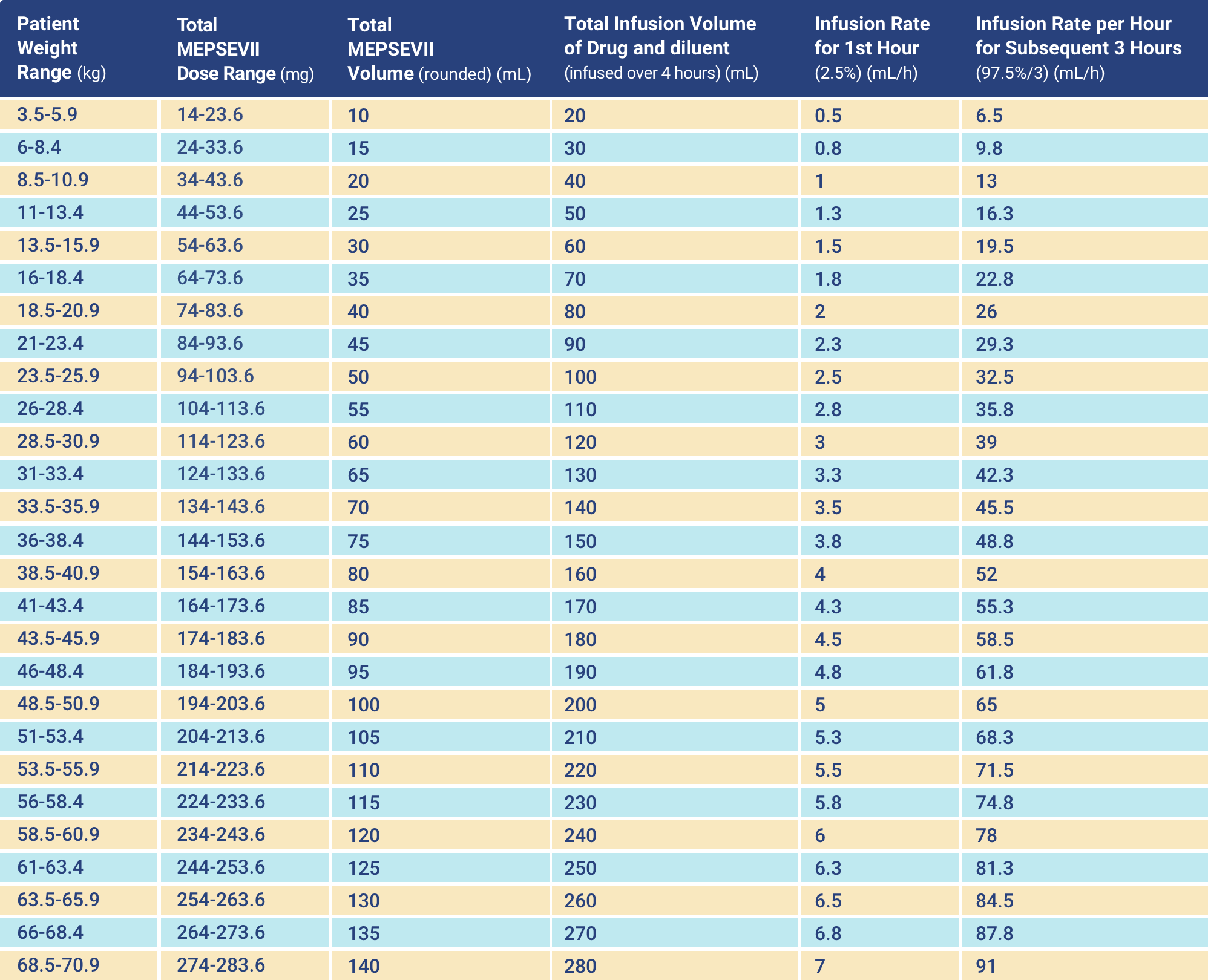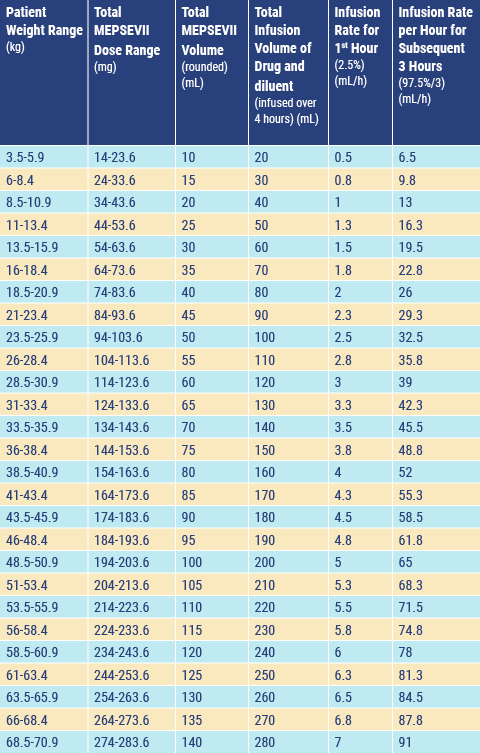MEPSEVII® (vestronidase alfa-vjbk) dosing chart

Recommended Infusion Rate Schedule by Patient Weight for Administration of MEPSEVII at Recommended Dose of 4 mg/kg

MEPSEVII® (vestronidase alfa-vjbk) dosing chart

Recommended Infusion Rate Schedule by Patient Weight for Administration of MEPSEVII at Recommended Dose of 4 mg/kgCalculating the Dose

• Weigh the patient to determine the number of vials to be diluted based on the patient’s weight and the recommended dose of 4 mg/kg
• Total dose (mg) = Patient’s weight (kg) x 4 mg/kg (recommended dose)
• Total number of vials = Total dose (mg) divided by 10 mg/vial
• Round to the next whole vial to determine the final total volume and number of vials needed based on the patient’s weight
• Volume (mL) of calculated dose = Total dose (mg) divided by the 2 mg/mL concentrationCalculating the Dose

• Weigh the patient to determine the number of vials to be diluted based on the patient’s weight and the recommended dose of 4 mg/kg
• Total dose (mg) = Patient’s weight (kg) x 4 mg/kg (recommended dose)
• Total number of vials = Total dose (mg) divided by 10 mg/vial
• Round to the next whole vial to determine the final total volume and number of vials needed based on the patient’s weight
• Volume (mL) of calculated dose = Total dose (mg) divided by the 2 mg/mL concentration